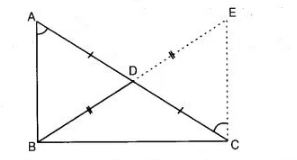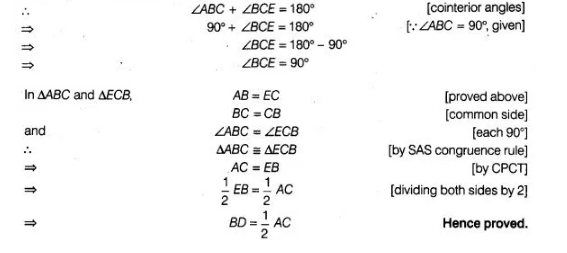# In a right triangle, prove that the line-segment`
Question:

In a right triangle, prove that the line-segment joining the mid-point of the hypotenuse to the opposite vertex is half the hypotenuse.

Solution:

Given In $\triangle A B C, \angle B=90^{\circ}$ and $D$ is the mid-point of $A C$.

Construction Produce $B D$ to $E$ such that $B D=D E$ and join $E C$.

To prove $B D=\frac{1}{2} A C$Proof in $\triangle A D B$ and $\triangle C D E$, $A D=D C$ $[\because D$ is mid-point of $A C]$

$B D=D E$ [by construction]

and $\angle A D B=\angle C D E$ [vertically opposite angles]

$\therefore$ $\triangle A D B \cong \triangle C D E$ [by SAS congruence rule]

$\Rightarrow$   $A B=E C$   [by CPCT]

and $\angle B A D=\angle D C E$ [by CPCT]

But $\angle B A D$ and $\angle D C E$ are alternate angles.

So, $E C \| A B$ and $B C$ is a transversal.